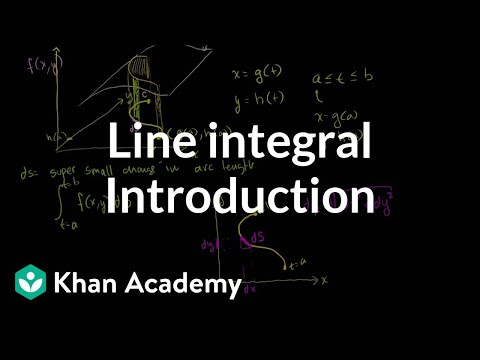### Calculus: Line integrals and Green's theorem

Free Closed [?]Line integral of scalar and vector-valued functions. Green's theorem and 2-D divergence theorem.
Introduction to the Line Integral. Line Integral Example 1. Line Integral Example 2 (part 1). Line Integral Example 2 (part 2). Position Vector Valued Functions. Derivative of a position vector valued function. Differential of a vector valued function. Vector valued function derivative example. Line Integrals and Vector Fields. Using a line integral to find the work done by a vector field example. Parametrization of a Reverse Path. Scalar Field Line Integral Independent of Path Direction. Vector Field Line Integrals Dependent on Path Direction. Path Independence for Line Integrals. Closed Curve Line Integrals of Conservative Vector Fields. Example of Closed Line Integral of Conservative Field. Second Example of Line Integral of Conservative Vector Field. Green's Theorem Proof Part 1. Green's Theorem Proof (part 2). Green's Theorem Example 1. Green's Theorem Example 2. Constructing a unit normal vector to a curve. 2 D Divergence Theorem. Conceptual clarification for 2-D Divergence Theorem. Introduction to the Line Integral. Line Integral Example 1. Line Integral Example 2 (part 1). Line Integral Example 2 (part 2). Position Vector Valued Functions. Derivative of a position vector valued function. Differential of a vector valued function. Vector valued function derivative example. Line Integrals and Vector Fields. Using a line integral to find the work done by a vector field example. Parametrization of a Reverse Path. Scalar Field Line Integral Independent of Path Direction. Vector Field Line Integrals Dependent on Path Direction. Path Independence for Line Integrals. Closed Curve Line Integrals of Conservative Vector Fields. Example of Closed Line Integral of Conservative Field. Second Example of Line Integral of Conservative Vector Field. Green's Theorem Proof Part 1. Green's Theorem Proof (part 2). Green's Theorem Example 1. Green's Theorem Example 2. Constructing a unit normal vector to a curve. 2 D Divergence Theorem. Conceptual clarification for 2-D Divergence Theorem.

Categories:

# Reviews

-- no reviews yet -- make the first review

# Alternatives All alternatives to all Khan Academy courses

-- no alternatives found for the course --
If you know any alternatives, please let us know.

# Prerequisites

-- no prerequsites found for the course --
If you can suggest any prerequisite, please let us know.

# Paths

No Paths inclusing the course. You can build and share a path with this course included.

# Certification Exams

-- there are no exams to get certification after this course --
If your company does certification for those who completed this course then register your company as certification vendor and add your exams to the Exams Directory.

# Similar courses

Courses related to the course subject

### Calculus I (Differential Calculus)

Videos on a first course in calculus (Differential Calculus).

### Calculus II (Integral Calculus)

Videos on a second course in calculus (Integral Calculus).

### Calculus One

Calculus One is a first introduction to differential and integral calculus, emphasizing engaging examples from everyday life.

Details:

### Calculus: AP Calculus practice questions

Sample questions from the A.P. Calculus AB and BC exams (both multiple choice and free answer). 2011 Calculus AB Free Response #1a…

### Calculus: Derivative applications

Minima, maxima, and critical points. Rates of change. Optimization. Rates of change. L'Hopital's rule. Mean value theo…

### Calculus: Divergence theorem

Divergence theorem intuition. Divergence theorem examples and proofs. Types of regions in 3D. 3-D Divergence Theorem Intuition.

### Calculus: Double and triple integrals

Volume under a surface with double integrals. Triple integrals as well. Double Integral 1. Double Integrals 2. Double Integrals 3.

### Calculus: Indefinite and definite integrals

Indefinite integral as anti-derivative. Definite integral as area under a curve. Integration by parts. U-substitution. Trig su…

### Calculus: Limits

Limit introduction, squeeze theorem, and epsilon-delta definition of limits. Introduction to limits. Limit at a point of discontin…

### Calculus: Line integrals and Green's theorem

Line integral of scalar and vector-valued functions. Green's theorem and 2-D divergence theorem. Introduction to the Line In…

Let us know when you did the course Calculus: Line integrals and Green's theorem.

 Started on: Completed on: Your grade (if any):Comments:Add the course Calculus: Line integrals and Green's theorem to My Personal Education Path.

 Start the course on: Duration of study: 1 Week 2 Weeks 3 Weeks 4 Weeks 5 Weeks 6 Weeks 7 Weeks 8 Weeks 10 Weeks 12 Weeks 16 Weeks Notes: